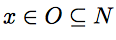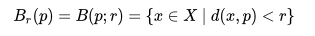# Deleted Neighborhood: Simple Definition, Examples

## What is a Neighborhood?

A neighborhood (or neighbourhood), in math, is very like the concept in everyday speech; It’s a defined space surrounding some point X.

## More Formal Definition in Topology

Although neighborhoods can be defined in many different ways, in topology it’s defined in terms of topological spaces.

For any topological space X and any point x in X, we can say that a neighborhood of p is a subset of X (call this subset N, for ‘neighborhood’) which includes an open set (call this one O, for ‘open’) which contains x.

In math, we’d write that like this:This doesn’t tell much about the size and shape of our neighbourhood, but that’s because there are many neighbourhoods, of different shapes and sizes, you could define for your point x. It does tell us that x is an interior point of it’s neighbourhood; it’s not on the edge.

Notice that our neighbourhood N also doesn’t have to be an open set; it just includes an open set. If the neighbourhood is itself an open set is called an open neighborhood.

The neighborhood of a set is very like the neighbourhood of a point. If S is a subset of a topological space X, then we say the neighbourhood of S is a set (call it N) which includes an open set U which contains S.

## A Neighborhood in a Metric Space

There’s a special definition of neighbourhoods for metric spaces as well. A metric space is a set (call it M) where a notion of distance is defined and satisfies some basic, specified conditions.

The definition of a neighbourhood on metric spaces states that in a n-dimensional metric space
M = (X, d),
a set N is a neighborhood of a point p if there is an open n-dimensional ball centered at p, with a radius larger than zero, for which the entire ball, as specified byis contained in the neighbourhood N.

## R-Neighborhoods

So far, our neighbourhoods might be any shape under the sun. They contain intervals; they contain balls, but they are under no uniformity requirement. Sometimes though we want nice neat neighborhoods that have symmetry and a little more order. That’s where r-neighborhoods come in.

An r-neighbourhood of S is the set of all points in X (where X is the metric space containing S) which are less than r-distance from the set S. In order for this to work, r has to be greater than zero. Sometimes, the r-neighborhood is defined as the union of all the open balls of radius r centered at the point S. This definition, though, is equivalent to the first definition.

## Deleted Neighborhood

A deleted neighborhood is the area (neighborhood) surrounding a specific point, minus the point itself. A neighborhood is basically a set of points (which contains the specific point you’re interested in) where you can move around without leaving the set.

Intuitively, the term seems to imply that it’s the neighborhood that’s deleted, rather than the point itself. But let’s say you imagine you’re inside your house, in your neighborhood. Your house disappears (with you inside it), and you’re transported to the middle of the desert. When you open your front door, you won’t think it’s your house that has disappeared—you’ll wonder what happened to the neighborhood.

## Definition with Real Numbers

A deleted neighborhood can be defined in terms of real numbers: it is an interval on the number line with the center point removed.

A neighborhood is always defined by two numbers: a point (z0) and a constant— any positive real number. That number is often symbolized by δ. Using those symbols, we can say that a neighborhood of z0 is the set of all points a such that the absolute value |z-z0| is less than δ.

Using that definition as a basis, a deleted neighborhood can be defined as the set which includes every point for which |z-z0| is greater than 0 and less than &delta.## Visualizing a Deleted Neighborhood

A deleted neighborhood is often called a punctured neighborhood. That gives a good visual way of understanding the meaning. Imagine, on a number line, you mark with your highlighter pen an area from z0 – δ to z0 + δ, and then imagine you take a pin and puncture the point z0 so it is no longer part of the colored bar,and then you will have a representation of a punctured neighborhood.

## Examples

In interval notation, a deleted neighborhood would be described as the set {x:|x-z0 | < δ }.

• {x: |x – 4| < 2}— the interval on the real number line between 2 and 6, with the point 4 taken out.
• {x: |x | < 5}— the interval centered around zero from -5 to 5, but the center point (0) is taken out.
• {x: |x – 5.9| < 0.1 }— the interval centered around 5.9 from 5.8 to 6.0, with the center point 5.9 taken out. This interval is graphed below:## References

Buckmire. Math 312 Complex Analysis Class Notes. Retrieved from https://sites.oxy.edu/ron/math/312/98/worksheets/05.pdf on November 16, 2018.
Cavalieri, Renzo. Renzo’s Math 490 Introduction to Topology. Retrieved from https://www.math.colostate.edu/~renzo/teaching/Topology10/Notes.pdf on October 22, 2019.